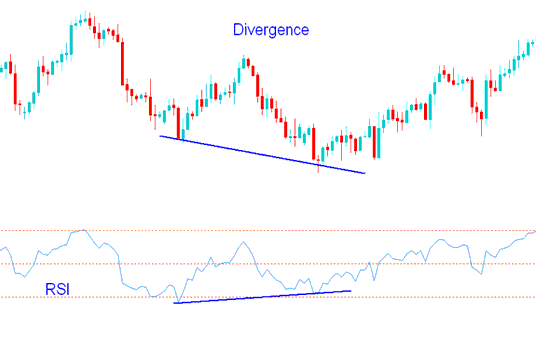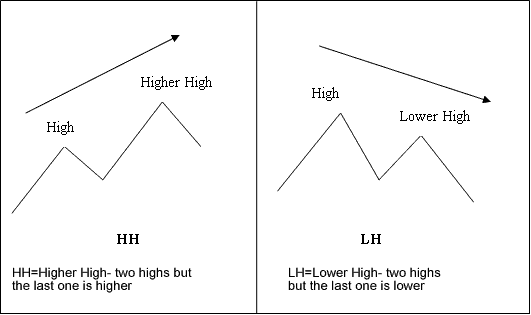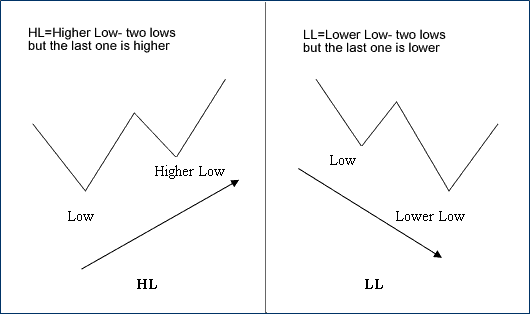# What is RSI Indices Divergence?

RSI Divergence Indices Trading is one of the indices trading strategy used by online stock indexes traders to analyze indices chart stock indexes price movement. RSI Indices Divergence involves looking at a stock indices chart and one more stock indexes technical indicator - RSI indicator. For our this stock indexes trading divergence tutorials we shall use the RSI indices indicator - RSI Stock Indexes Divergence.

To spot this RSI indices divergence trading setup find two indices chart points at which stock indexes price makes a new swing high or a new swing low but the RSI stock indices indicator does not do the same, thus indicating that there is a divergence between the stock indexes price and momentum.

In the stock indices chart below we identify two indices chart points, chart point A and chart point B (swing highs)

Then using RSI stock indices indicator we check the highs made by the RSI indices indicator, these are the highs that are directly below the Chart points marked A and B.

We then draw one line on the stock indices chart and another line on the RSI stock indices indicator.RSI Indices Divergence Meaning - RSI Indices Trading Divergence Tutorial - RSI Divergence Indices Trading Strategy PDF - RSI Divergence PDF

How to trade RSI indices divergence

In order to trade RSI indices divergence setup we look for the following setups:

HH - Higher High - two highs but the last one is higher

LH - Lower High - two highs but the last one is lower

HL - Higher Low - two lows but the last one is higher

LL - Lower Low - two lows but the last one is lower

First let us look at the examples of these indices divergence trading termsIndices RSI Divergence Meaning - Indices RSI Divergence TutorialRSI Stock Indexes Divergence Definition - RSI Divergence Indices Trading Tutorial - RSI Indices Trading Divergence Strategy PDF - RSI Divergence Indices Trading PDF

There are two types of indices trading RSI divergence setups:

1. RSI Classic Divergence

2. RSI Hidden Divergence